﻿ 面向流域的定量降水估测产品检验订正
 快速检索 中文标题 英文标题 作者中文名 作者英文名 单位中文名 单位英文名 中文关键词 英文关键词 中文摘要 英文摘要 基金项目 全文
 气象2016, Vol. 42Issue (10): 1245-1255.  DOI: 10.7519/j.issn.1000-0526.2016.10.009引用本文 [复制中英文]

[复制中文]
XU Fengwen, WANG Zhi, DI Jingyue, et al, 2016. Verification and Correction of Quantitative Precipitation Estimation for River Basins[J]. Meteorological Monthly, 42(10): 1245-1255. DOI: 10.7519/j.issn.1000-0526.2016.10.009.
[复制英文]

文章历史

2015年6月06日收稿
2016年8月17日收修定稿

1. 国家气象中心, 北京 100081
2. 中国气象局公共气象服务中心, 北京 100081
3. 中国气象科学研究院灾害天气国家重点实验室, 北京 100081

Verification and Correction of Quantitative Precipitation Estimation for River Basins
XU Fengwen1,2, WANG Zhi2, DI Jingyue1,2, BAO Hongjun1,2, ZHAO Linna3
1. National Meteorological Centre, Beijing 100081;
2. Public Meteorological Service Centre, CMA, Beijing 100081;
3. State Key Laboratory of Severe Weather, Chinese Academy of Meteorological Sciences, Beijing 100081
Abstract: With the development of fusion technology of multi-resource precipitation data, quantitative precipitation estimation (QPE) that combines satellite, radar and conventional meteorological data has got more mature. It has effectively made up the deficienties of low spatial and temporal resolution of conventional meteorological data and offered good opportunities for the application and development of the watershed area precipitation products. At the same time, the testing and the correction of suitability of QPE are the premise and basis for the application of watershed area precipitation. In the paper, based on hydrological precipitation data, statistic-testing methods such as the threat score (TS), accuracy rate, false-positives rate, false-negatives rate, absolute bias, relative bias, root mean square error and the ROC curve are employed to evaluate the suitability of the QPE of National Meteorological Centre. The error spatial and temporal distribution, the series error in river basin and the quality of different scale precipitation are analyzed. Then, the decreasing average method is applied to optimize and correct the QPE which is based on the error statistics. The watershed area precipitation products are calculated based on the optimized QPE. Lastly, in the case of hydrological element forecast in Linyi Basin, the QPE area precipitation products are found good for the improvement of hydrological forecast. The studies indicate that developing QPE area precipitation products based on fusion technology of multi-resource precipitation data can make up the deficiencies of refinement in state-level forecasting operation of area precipitation and, enhance the technological capacity of state-level hydrological meteorology.
Key words: QPE (quantitative precipitation estimation)    watershed area precipitation    test

1 研究区域和数据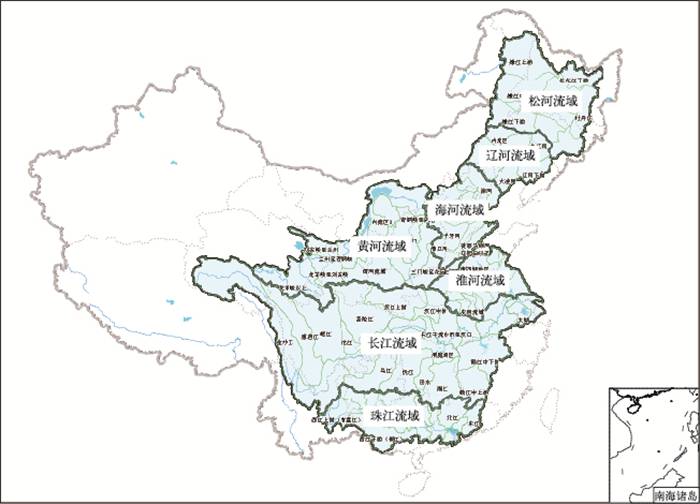图 1 全国七大江河流域示意图 Fig. 1 Sketch map of seven major river basins in China

2 面向流域定量估测降水检验 2.1 检验方法

 $绝对误差:{\mathit{X}_\mathit{a}}{\rm{ = }}\sum\limits_{\mathit{i}{\rm{ = 1}}}^\mathit{N} {{\rm{|}}{\mathit{X}_{\rm{f}}}{\rm{ - }}{\mathit{X}_{\rm{o}}}{{\rm{|}}_\mathit{i}}} {\rm{ }}$ (1)
 $相对误差:{\mathit{X}_\mathit{b}}{\rm{ = }}{\mathit{X}_\mathit{a}}{\rm{/}}{\mathit{X}_{\rm{o}}}$ (2)
 $均方根误差:{{\mathit{X}}_{\mathit{c}}}\rm{=}{{\left[ \frac{1}{\mathit{N}}\sum\limits_{\mathit{i}\rm{=1}}^{\mathit{N}}{\rm{(}{{\mathit{X}}_{\rm{f}}}\rm{-}{{\mathit{X}}_{\rm{o}}}\rm{)}_{\mathit{i}}^{2}} \right]}^{1/2}}\rm{ }\!\!~\!\!\rm{ }$ (3)
 $平均误差{\rm{:}}{\mathit{X}_\mathit{d}}{\rm{ = }}\frac{1}{\mathit{N}}\sum\limits_{\mathit{i}{\rm{ = 1}}}^\mathit{N} {{{{\rm{(}}{\mathit{X}_{\rm{f}}}{\rm{ - }}{\mathit{X}_{\rm{o}}}{\rm{)}}}_\mathit{i}}}$ (4)

 $\mathit{TS} = {\mathit{N}_\mathit{A}}/({\mathit{N}_\mathit{A}} + {\mathit{N}_\mathit{B}} + {\mathit{N}_\mathit{C}})$ (5)

 $漏估率:\mathit{PO} = {\mathit{N}_\mathit{C}}/({\mathit{N}_\mathit{A}} + {\mathit{N}_\mathit{C}})$ (6)
 $空估率:\mathit{FAR} = {\mathit{N}_\mathit{B}}/({\mathit{N}_\mathit{A}} + {\mathit{N}_\mathit{B}})$ (7)
 $估测偏差:\mathit{BIAS} = ({\mathit{N}_\mathit{A}} + {\mathit{N}_\mathit{B}})/({\mathit{N}_\mathit{A}} + {\mathit{N}_\mathit{C}})$ (8)
 $命中率:\mathit{POD} = {\mathit{N}_\mathit{A}}/({\mathit{N}_\mathit{A}} + {\mathit{N}_\mathit{C}})$ (9)

 $\begin{array}{l} 真阳性率(TPR)即命中率:\\ \;\;\;\;\;\;\;\;\;\;\;\;\;\mathit{TPR} = {\mathit{N}_\mathit{A}}/({\mathit{N}_\mathit{A}} + {\mathit{N}_\mathit{C}}) \end{array}$ (10)
 $\begin{array}{l} 假阳性率(FPR)即错误命中率:\;\;\;\;\;\;\;\;\;\;\;\;\\ \;\;\;\;\;\;\;\;\;\;\;\;\;\;\mathit{FPR} = {\mathit{N}_\mathit{B}}/({\mathit{N}_\mathit{B}} + {\mathit{N}_\mathit{D}}) \end{array}$ (11)
2.2 检验结果 2.2.1 QPE1检验结果

2012和2013年汛期(5—9月)306 d的七大流域时空平均QPE1产品检验结果表明(表 1)，七大流域的均方根误差在5.86~12.17，其中长江流域误差值＞10，珠江流域也接近10，估测降水在这两个流域上误差较大，而QPE1产品对于黄河、辽河流域和松花江流域的估测能力略强，其均方根误差较小；估测降水的绝对误差2.97~5.33，与均方根误差对应的是，其在长江流域和珠江流域误差较大；估测降水平均误差普遍小于0，说明QPE1估测降水值普遍比实况值偏小；降水估测相对误差为1.13~1.84，相对误差平均值1.49，在各大流域误差相当。从这四个指标综合来看，对于整个样本检验结果在我国偏北的辽河、黄河等流域的估测效果较好，而对于偏南的长江、珠江效果相对差一些。我国南北方差异大，特别是汛期，南方平均日降水量大，降水范围广，尤其长江和珠江流域整个汛期一直处于强降雨带中，北方则平均日降水量少，由此可知，QPE1对降水量级大的区域估测能力相对较弱。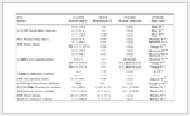表 1 2012和2013年的5—9月面向七大流域的QPE1降水检验评估 Table 1 QPE1 precipitation test and evaluation for the seven major river basins from May to September in 2012 and 2013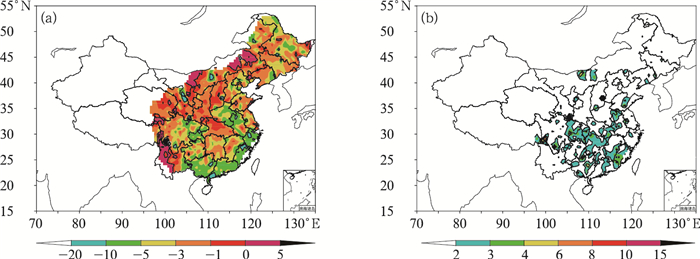图 2 2012和2013年的5月1日至9月30日七大流域QPE1平均误差(a，单位：mm)和相对误差(b，单位：%)的空间分布 Fig. 2 Distribution of the average error (a, unit:mm) and relative error (b, unit:%) of QPE1 for the seven major river basins from 1 May to 30 September in 2012 and 2013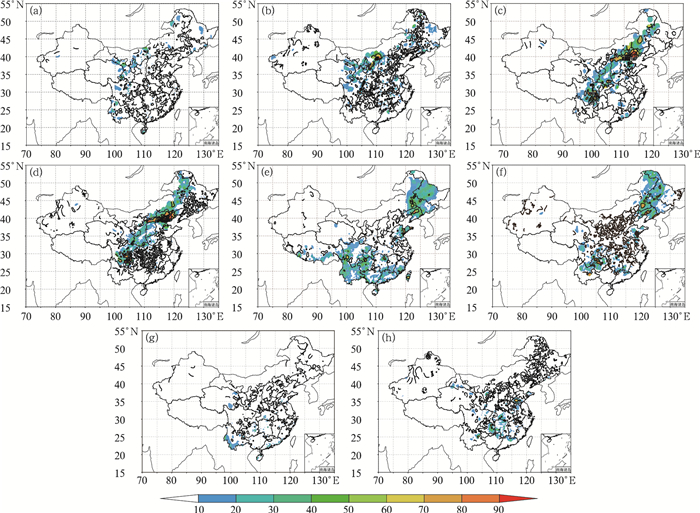图 3 QPE1和水文站观测降水量(单位：mm)对比图(a, c, e, g)7月20—23日QPE1；(b, d, f, h)水文站7月20—23日观测降水 Fig. 3 Comparison of precipitation (unit: mm) of hydrological stations and QPE1 (a, c, e, g) QPE1 in 20-23 July, (b, d, f, h) precipitation of hydrological station 20-23 July
2.2.2 QPE1和QPE2检验评估对比分析表 2 2012和2013年5月1日至9月30日QPE1和QPE2检验结果对比 Table 2 Test results of QPE1 and QPE2 for different grades of precipitation from 1 May to 30 September in 2012 and 2013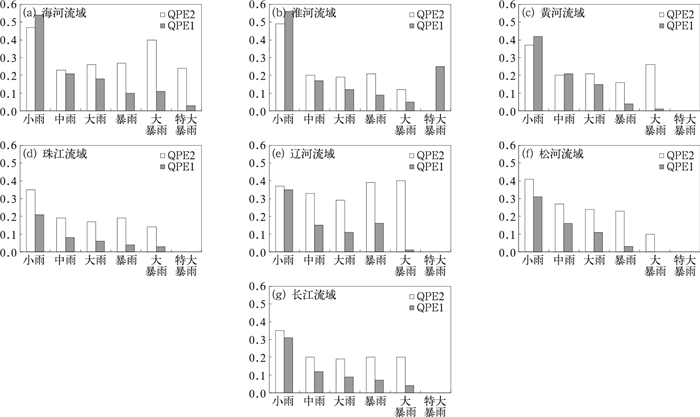图 4 2012和2013年5月1日至9月30日七大流域QPE1和QPE2的TS评分 Fig. 4 TS score of QPE1 and QPE2 for the seven major river basins from 1 May to 30 September in 2012 and 2013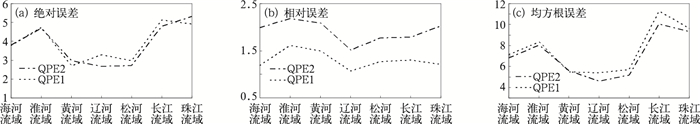图 5 2012和2013年5月1日至9月30日七大流域QPE1和QPE2估测降水的绝对误差(a)、相对误差(b)和均方根误差(c) Fig. 5 Absolute error (a), relative error (b) and root mean squared error (c) of QPE1 and QPE2 for the seven major river basins from 1 May to 30 September in 2012 and 2013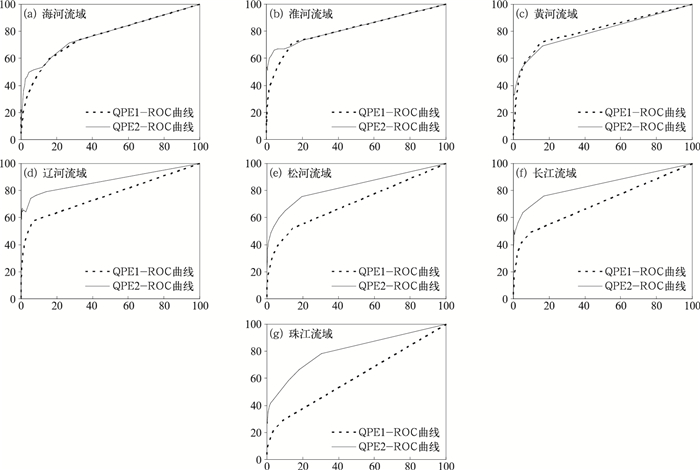图 6 2012和2013年的5月1日至9月30日七大流域QPE1和QPE2 ROC曲线 Fig. 6 ROC curve of QPE1 and QPE2 for the seven major rivers from 1 May to 30 September in 2012 and 2013
3 面向流域定量估测降水订正 3.1 订正方法

(1) 误差估计。计算QPE对于实况降水的误差，记为bi, j，表示如下：

 ${\mathit{b}_{\mathit{i}{\rm{, }}\mathit{j}}}\left(\mathit{t} \right) = {\mathit{f}_{\mathit{i}{\rm{, }}\mathit{j}}}\left(\mathit{t} \right) - {\mathit{o}_{\mathit{i}{\rm{, }}\mathit{j}}}\left(\mathit{t} \right)$ (12)

(2) 误差累加。将最新的各个时次估测误差累加到上一个时次的误差场，得到更新后的误差场。

 ${\mathit{B}_{\mathit{i}{\rm{, }}\mathit{j}}}\left(\mathit{t} \right) = \left({1 - \mathit{w}} \right){\mathit{B}_{\mathit{i}{\rm{, }}\mathit{j}}}\left({\mathit{t} - 2} \right) + \mathit{w}{\mathit{b}_{\mathit{i}{\rm{, }}\mathit{j}}}\left({\mathit{t} - 1} \right)$ (13)

(3) 误差订正。将当日的QPE减去新的误差场得到最终的订正场。

 ${\mathit{F}_{\mathit{i}{\rm{, }}\mathit{j}}}\left(\mathit{t} \right) = {\mathit{f}_{\mathit{i}{\rm{, }}\mathit{j}}}\left(\mathit{t} \right) - {\mathit{B}_{\mathit{i}{\rm{, }}\mathit{j}}}\left(\mathit{t} \right)$ (14)

3.2 面向流域的QPE1产品订正结果 3.2.1 2013年QPE1订正权重的确定表 3 订正后的误差平均、相对误差平均和均方根误差平均 Table 3 Absolute error, relative error and root mean squared error after correction

3.2.2 2013年QPE1订正结果检验分析表 4 QPE1订正前后的误差对比 Table 4 Error analysis of original QPE1 and corrected QPE1表 5 QPE1订正前后不同降水量级相对误差对比 Table 5 Error analysis of original QPE1 and corrected QPE1 based on different rainfall grades
3.2.3 2013年QPE1订正结果检验参数空间分布对比分析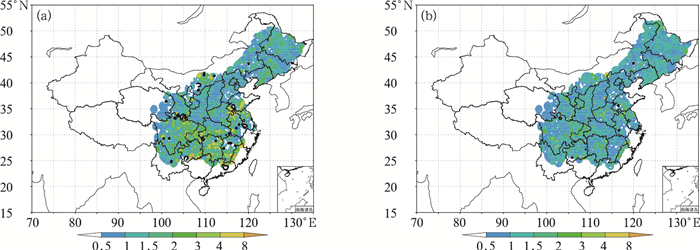图 7 2013年订正前(a)和订正后(b)QPE1相对误差的全国分布图 Fig. 7 Spatial distribution of original (a) and corrected (b) QPE1 relative error in 2013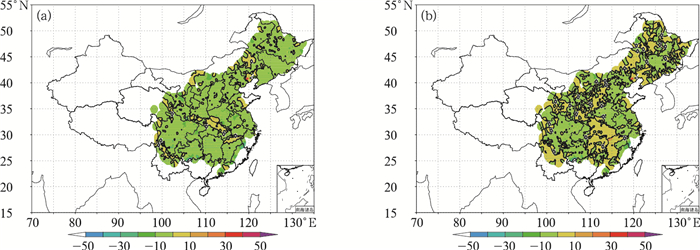图 8 同图 7，但为误差平均(单位：mm) Fig. 8 Same as Fig. 7, but for average error (unit:mm)
3.2.4 2012年QPE1订正结果检验分析

4 基于定量估测降水的面雨量在水文预报中的个例检验 4.1 试验流域概况

4.2 基于订正前后的QPE1面雨量在水文预报中的检验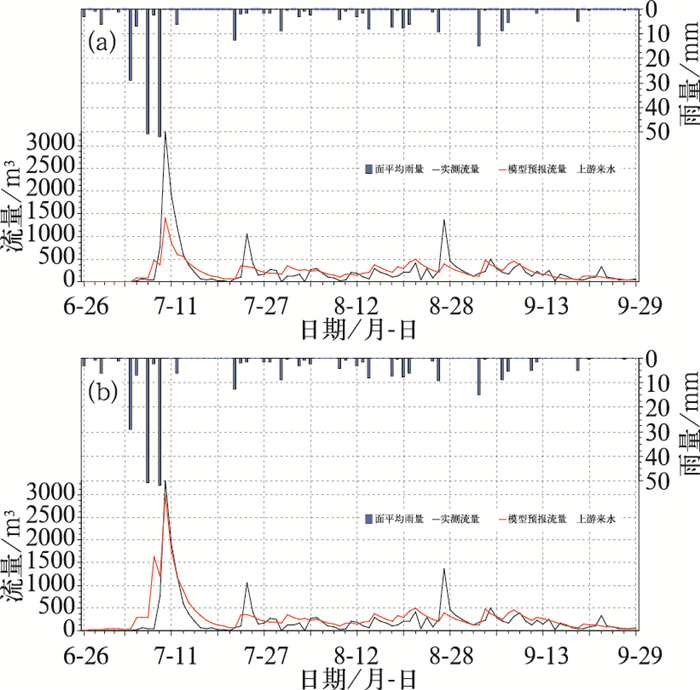图 9 基于QPE1的面雨量在2012年流域水文预报中的预报结果 (a)面雨量在2012年流域水文预报中的预报结果，(b)订正面雨量在2012年流域水文预报中的预报结果 Fig. 9 Forecasts of area precipitation based on QPE1 in the 2012 river basin hydrological forecasts (a) original area precipitation, (b) corrected area precipitation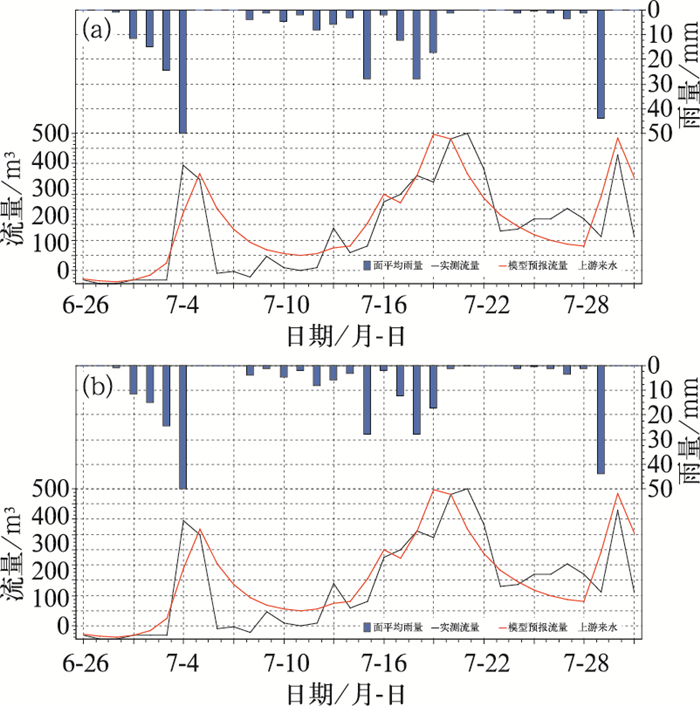图 10 同图 9，但为2013年 Fig. 10 Same as Fig. 9, but for 2013
 ${\mathit{D}_\mathit{c}} = \frac{{{{\sum\limits_{\mathit{i} = 1}^\mathit{n} {\left[ {{\mathit{y}_\mathit{c}}\left(\mathit{i} \right) - {\mathit{y}_{\rm{0}}}\left(\mathit{i} \right)} \right]} }^2}}}{{\sum\limits_{\mathit{i} = 1}^\mathit{n} {{{\left[ {{\mathit{y}_\mathit{c}}\left(\mathit{i} \right) - {{\bar y}_{\rm{0}}}} \right]}^2}} }}$

5 结论及成果应用前景

(1) 针对国家级QPE产品进行适用性评估。融合自动站雨量计降水及卫星、雷达等反演的降水产品有效利用了地面观测和反演降水各自的优势，在降水量值和空间分布上均较为合理，对10 mm以下降水有较好的估测能力，降水值偏大的时候，估测效果明显下降。其次，该产品的估测降水值相比水文站观测降水整体呈偏小趋势。另外，在黄河流域、松花江流域及珠江等流域中，该产品的估测能力较好，其余流域次之。基于TS评分、漏估率、空估率、估测偏差BIAS、命中率等指标，融合自动站雨量计降水、卫星、雷达反演的降水产品估测评分效果普遍低于仅融合自动站雨量计降水、卫星反演的降水产品估测评分值。

(2) 针对国家气象中心QPE产品，运用“递减平均法”对2013年进行了订正试验，确定在不考虑2012年误差平均的基础上，采用0.8的订正系数时，订正效果相对较好，订正后2013年QPE误差平均绝对值和相对误差均有不同程度的减小，均方根误差有一定的增大。订正后，大雨以下量级的相对误差改善较为明显，而大雨以上量级效果改善不明显。对2012年的订正权重系数为0.7时，订正效果较优，对于连续性降水，订正后的QPE在降水的连续性和量值分布上都较订正前有明显的提高。但对于降水仅出现在24 h内的降水，订正效果并不明显

(3) 通过应用“基于QPE的江河流域面雨量精细化计算系统”，将QPE产品转化为适于水文气象服务的江河流域面雨量产品，在沂河临沂站以上流域的两次连续性降水过程开展水文模拟试验表明，两次过程均与实际流量过程较为吻合，但订正前后的效果并不明显，主要原因是大雨量级的面雨量计算订正效果较差，小量级面雨量改善并不能对水文模拟起到关键作用。

(4) 本研究是对国家级QPE产品的面向流域的运用开发，首次对比了两种QPE产品的性能。研究开发了基于QPE产品的江河流域面雨量精细化计算系统，实现了QPE在水文预报的应用，弥补了过去面雨量计算主要靠空间插值的多种不足。

 陈丽娟, 张培群, 赵振国, 2005. 松嫩辽流域夏季面雨量预测因子探讨[J]. 应用气象学报, 16(5): 663-669. DOI:10.11898/1001-7313.20050513 程丛兰, 陈明轩, 王建捷, 等, 2013. 基于雷达外推临近预报和中尺度数值预报融合技术的短时定量降水预报试验[J]. 气象学报, 71(3): 397-415. DOI:10.11676/qxxb2013.041 方慈安, 潘志祥, 叶成志, 等, 2003. 几种流域面雨量计算方法的比较[J]. 气象, 28(7): 23-26. DOI:10.7519/j.issn.1000-0526.2003.07.005 管成功, 王克敏, 陈晓红, 2006. 2002—2005年T213数值降水预报产品分析检验[J]. 气象, 32(8): 70-76. DOI:10.7519/j.issn.1000-0526.2006.08.012 李俊, 支树林, 郭艳, 等, 2012. SWAN系统雷达定量降水估测产品在江西的应用[J]. 气象与减灾研究, 35(2): 61-66. 李莉, 李应林, 田华, 等, 2011. T213全球集合预报系统性误差订正研究[J]. 气象, 37(1): 31-38. DOI:10.7519/j.issn.1000-0526.2011.01.004 刘静, 叶金印, 张晓红, 等, 2012. 2011年6—8月淮河流域面雨量预报检验[J]. 安徽农业科学, 40(36): 17698-17700. DOI:10.3969/j.issn.0517-6611.2012.36.097 吕晓娜, 牛淑贞, 袁春风, 等, 2013. SWAN中定量降水估测和预报产品的检验与误差分析[J]. 暴雨灾害, 32(2): 142-150. 邱学兴, 王东勇, 陈宝峰, 2012. T639模式预报系统误差统计和订正方法研究[J]. 气象, 38(5): 526-532. DOI:10.7519/j.issn.1000-0526.2012.05.002 沈艳, 潘畅, 宇婧婧, 等, 2013. 中国区域小时降水量融合产品的质量评估[J]. 大气科学学报, 36(1): 37-46. 田付友, 程明虎, 张亚萍, 等, 2010. 校准雨量计密度对雷达联合雨量计估测流域平均面雨量的影响[J]. 气象学报, 68(5): 718-730. 王运生, 谢丙炎, 万方浩, 等, 2007. ROC曲线分析在评价入侵物种分布模型中的应用[J]. 生物多样性, 15(4): 365-372. 肖丹, 邓莲堂, 陈静, 等, 2010. T213与T639资料驱动WRF的预报初步检验比较[J]. 暴雨灾害, 29(1): 20-29. 徐晶, 林建, 姚学祥, 等, 2001. 七大江河流域面雨量计算方法及应用[J]. 气象, 27(11): 13-16. DOI:10.3969/j.issn.1000-0526.2001.11.003 徐晶, 姚学祥, 2007. 流域面雨量估算技术综述[J]. 气象, 33(7): 15-21. DOI:10.7519/j.issn.1000-0526.2007.07.002 翟丹华, 张亚萍, 邱鹏, 等, 2015. 琼江两次洪水过程的水文气象分析[J]. 气象, 41(1): 59-67. DOI:10.7522/j.issn.1000-0534.2013.00156 张国平, 赵琳娜, 许凤雯, 等, 2010. 基于流域结构分析的中国流域划分方案[J]. 北京师范大学学报(自然科学版), 46(3): 417-423. 张亚萍, 程明虎, 徐慧, 等, 2007. 雷达定量测量降水在佛子岭流域径流模拟中的应用[J]. 应用气象学报, 18(3): 295-305. 张亚萍, 张勇, 廖峻, 等, 2013. 天气雷达定量降水估测不同校准方法的比较与应用[J]. 气象, 39(7): 923-929. DOI:10.7519/j.issn.1000-0526.2013.07.013 赵琳娜, 吴昊, 田付友, 等, 2010. 基于TIGGE资料的流域概率性降水预报评估[J]. 气象, 36(7): 133-142. DOI:10.7519/j.issn.1000-0526.2010.07.020